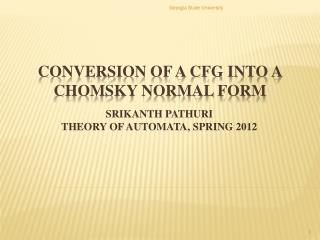# CONVERSION OF A CFG INTO A CHOMSKY NORMAL FORM - PowerPoint PPT PresentationDownload PresentationCONVERSION OF A CFG INTO A CHOMSKY NORMAL FORM

CONVERSION OF A CFG INTO A CHOMSKY NORMAL FORMDownload Presentation## CONVERSION OF A CFG INTO A CHOMSKY NORMAL FORM

- - - - - - - - - - - - - - - - - - - - - - - - - - - E N D - - - - - - - - - - - - - - - - - - - - - - - - - - -
##### Presentation Transcript

1. Georgia State University SRIKANTH PATHURITHEORY OF AUTOMATA, SPRING 2012 CONVERSION OF A CFG INTO A CHOMSKY NORMAL FORM

2. Definition: A CFG is said to be in Chomsky • normal form if every production is of one of these two types: • A->BC (where B and C are variables) • A-> σ(where σis a terminal) Georgia State University CHOMSKY Normal form

3. Georgia State University STEPS TO ACHIEVE CHOMSKY normal form

4. Let G be a CFG with the following Productions: Georgia State University Example Input Productions S->TU|V T->aTb|л U->cU|л V->aVc|W W->bW|л

5. Nullable Variables T U W V Z Eliminating Nullable variables S-> TU | T | U | V T -> aTb | ab U -> cU | c V -> aVc | ac | W W -> bW | b Georgia State University Step – I Removing the λ- Productions Input productions S->TU|V T->aTb|л U->cU|л V->aVc|W W->bW|л • Rule 1 : For every production A-> α in P, add to P1 every production obtained by deleting from α one or more variable‐occurrences involving a nullable variable. • Rule 2 : Delete every λ ‐production from P1, as well as every production of the form • A->A.

6. Below are the steps to remove the unit productions • Find out the A- derivable variables before applying rule 1 and rule 2. • If A->B is a production, then B is A-derivable. • If C is A‐derivable and C->B is a production, then B is A‐derivable. • Rule 1 : For every pair (A, B) where B is A- derivable and every non-unit production B->α, add the production A-> αto P1. • Rule 2 : Delete all unit productions from P1. Georgia State University Step – II Removing unit Productions

7. The A-derivable variables from the given example are • S‐derivable: • T : Due to S->T production. • U : Due to S->U production. • V : Due to S->V production. • W : Due to S->V and V->W production. • V‐derivable: • W : Due to V->W production. Georgia State University Applying Step – II to the example

8. S -> TU | T | U | V This is the resultant production after Step I. S-> TU | T | U | V|aTb | ab | cU | c | aVc | ac | bW | b This is the resultant production after applying Rule 1 of Step II. S->TU | T | U | V|aTb | ab | cU | c | aVc | ac | bW | b This is the resultant production after applying Rule 2 of Step II. S ->TU | aTb | ab | cU | c | aVc | ac | bW | b This is the resultant production after Step II. Georgia State University Applying Step – II to the example

9. Similarly the other Productions after removing the unit productions are: T -> aTb | ab U -> cU | c V -> aVc | ac | bW | b W -> bW | b Georgia State University Applying Step – II to the example

10. After applying the two rules of Step 3. The new productions are as follows. S -> TU | XaTXb | XaXb | XcU | c | XaVXc | XaXc | XbW | b T -> XaTXb | XaXb U ->X cU | c V -> XaVXc | XaXc | XbW | b W -> XbW | b Georgia State University STEP – III Removing the Terminal Symbols Input from Step II S ->TU | aTb | ab | cU | c | aVc | ac | bW | b T -> aTb | ab U-> cU | c V-> aVc | ac | bW | b W-> bW | b Rule 1 : Introduce for every terminal symbol σ a new variable Xσand production Xσ-> σ. Rule 2 : In every production whose right side has at least two symbols, replace every terminal by its new variable

11. After applying the two rules of Step 3. The new productions are as follows. S-> TU | XaY1 | XaXb | XcU | c | XaY2 | XaXc| XbW| b Y1 -> TXb ;Y2-> VXc T -> XaY3 | XaXb ;Y3 -> TXb U -> XcU | c ;V -> XaY4 | XaXc | XbW| b Y4 -> VXc ;W -> XbW| b Georgia State University Step – iv Conversion into Chomsky normal form Input from Step III S -> TU | XaTXb | XaXb | XcU | c | XaVXc | XaXc | XbW | b T -> XaTXb | XaXb U ->X cU | c V -> XaVXc | XaXc | XbW | b W -> XbW | b Rule 1:Replace a production like A->BACB by the productions A->BY1,Y1->AY2, Y2->CB, where Y1 and Y2 are new variables.

12. THANK YOU Georgia State University